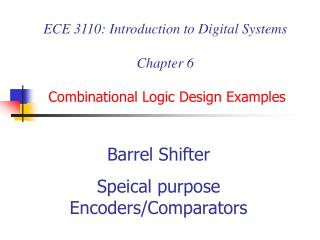# ECE 3110: Introduction to Digital Systems Chapter 6 Combinational Logic Design Examples - PowerPoint PPT PresentationDownload PresentationECE 3110: Introduction to Digital Systems Chapter 6 Combinational Logic Design Examples

ECE 3110: Introduction to Digital Systems Chapter 6 Combinational Logic Design ExamplesDownload Presentation## ECE 3110: Introduction to Digital Systems Chapter 6 Combinational Logic Design Examples

- - - - - - - - - - - - - - - - - - - - - - - - - - - E N D - - - - - - - - - - - - - - - - - - - - - - - - - - -
##### Presentation Transcript

1. ECE 3110: Introduction to Digital SystemsChapter 6Combinational Logic Design Examples Barrel Shifter Speical purpose Encoders/Comparators

2. 1 0 0 1 0 1

3. HW solutions Ex. 5.46 Ex. 5.19

4. Design examples using combinational building blocks (decoders, encoders, multiplexers, comparators, three-state devices, adders). • Barrel shifter • Dual-priority encoder • Cascading comparators, mode-dependent comparator

5. Barrel shifter design example • n data inputs, n data outputs • Control inputs specify number of positions to rotate or shift data inputs • Example: n = 16 • DIN[15:0], DOUT[15:0], S[3:0] (shift amount) • Many possible solutions, all based on multiplexers

6. Multiplexers (mux) Eg. Put between Processor’s registers and ALU A 16-bit processor where 3-bit field specifies on of 8 registers. The 3-bit field is connected to the select inputs of an 8-input, 16-bit mux. Select one of n sources of data to transmit on a bus.

7. MSI: 74x1518-input 1-bit multiplexer

8. 16-to-1 barrel shifter 16-to-1 mux = 2 x 74x151 8-to-1 mux + NAND gate

9. 4 16-bit 2-to-1 muxes 16-bit 2-to-1 mux = 4 x 74x157 4-bit 2-to-1 mux

10. Properties of different approaches

11. Dual-Priority Encoder • A priority encoder identifies not only the highest but also the second-highest-priority asserted signal among a set of 8 request inputs.

12. Single-priority encoder 0 1 0 0 1 0 0 1

13. 0 1 0 0 1 0 0 1

14. Parallel Cascading Comparators • 74x85: serial cascading scheme, delay of propagating the cascading signals through a cascade of comparators. • 74x682: Parallel approach, used to build very wide comparators. • Build 24-bit comparator, using 3 74x682 • PEQQ=EQ2.EQ1.EQ0 • PGTQ=GT2+EQ2.GT1+EQ2.EQ1.GT0

15. 8 bit Comparator +5V 74x85 74x85 A<B A<BIN A<BOUT A<BIN A<BOUT A=B A=BIN A=B OUT A=BIN A=B OUT A>B A>BIN A>BOUT A>BIN A>BOUT A0 A0 A4 A0 B0 B0 B4 B0 A1 A1 A5 A1 B1 B1 B5 B1 A2 A2 A6 A2 B2 B2 B6 B2 A3 A3 A7 A3 B3 B3 B7 B3 Least Significant bits Most Significant bits

16. Mode-dependent Comparator • Design a c.c. whose inputs are two 8-bit unsigned binary integers(X,Y), and a control signal(MIN/MAX). The output is an 8-bit unsigned binary integer Z, such that • Z=min(X,Y) if MIN/MAX=1; • Z=max(X,Y) otherwise

17. Next… • Sequential Logic Introduction